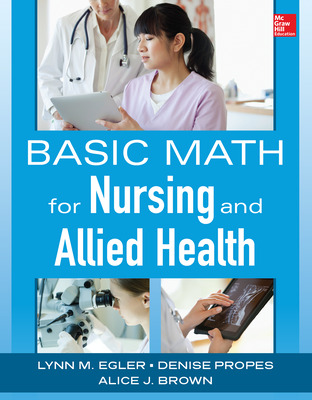# Basic Math for Nursing and Allied Health

1st Edition
By Lynn Egler and Denise Propes and Alice Brown
ISBN10: 0071829075
ISBN13: 9780071829076

## Purchase Options

Students, we’re committed to providing you with high-value course solutions backed by great service and a team that cares about your success. See tabs below to explore options and pricing. Don't forget, we accept financial aid and scholarship funds in the form of credit or debit cards.

### Product

Out of stock

The estimated amount of time this product will be on the market is based on a number of factors, including faculty input to instructional design and the prior revision cycle and updates to academic research-which typically results in a revision cycle ranging from every two to four years for this product. Pricing subject to change at any time.

### Program Details

CHAPTER 1 Understanding Numbers, Story Problems, and Numbering Systems
Introduction
Story Problems
Identifying and Extracting Information
Setting Up and Solving Equations
Arabic Numbers
Number Values
Combining and Counting
Roman Numerals
Rules for Roman Numerals
Combining Roman Numerals
Chapter Summary
Chapter Practice Test
CHAPTER 2 Basic Operations and Whole Numbers
Introduction
Basics of Subtraction
Subtracting Whole Numbers
Basics of Multiplication
Multiplication Tables
Multiplying Whole Numbers
Basics of Division
Dividing Whole Numbers
Chapter Summary
Chapter Practice Test
CHAPTER 3 Fractions and Mixed Numbers
Introduction
Fractions
Proper Fractions
Improper Fractions
Mixed Numbers
Reducing Fractions to Lowest Terms
Chapter Summary
Chapter Practice Test
CHAPTER 4 Basic Operations with Fractions
Introduction
Adding and Subtracting Fractions with Common Denominators
Adding and Subtracting Fractions with Different Denominators
Adding or Subtracting Fractions and Mixed or Whole Numbers
Multiplying Fractions and Mixed Numbers
Dividing Fractions and Mixed Numbers
Chapter Summary
Chapter Practice Test
CHAPTER 5 Working with Decimals
Introduction
Decimals
Converting Fractions to Decimals
Converting Decimals to Fractions
Rounding
Chapter Summary
Chapter Practice Test
CHAPTER 6 Basic Operations with Decimals
Introduction
Multiplying and Dividing Decimals
Chapter Summary
Chapter Practice Test
CHAPTER 7 Percents, Ratios, and Proportions
Introduction
Converting Decimals and Percents
Ratios and Proportions
Proportions
Cross Multiplication
Chapter Summary
Chapter Practice Test
CHAPTER 8 Systems of Measurement
Introduction
Working with Conversions
Standard Household System of Measurement
Metric System of Measurement
Converting Between Standard Household and Metric Systems of Measurements
Fraction Proportion Method
Ratio Proportion Method
Fraction Proportion Method
Ratio Proportion Method
Temperature
Time
Chapter Summary
Chapter Practice Test
CHAPTER 9 Putting It All Together
Introduction
Understanding Numbers, Story Problems, and Numbering Systems
Basic Operations and Whole Numbers
Fractions and Mixed Numbers
Basic Operations with Fractions
Working with Decimals
Basic Operations with Decimals
Percents, Ratios, and Proportions
Systems of Measurement
Comprehensive Exam (Chapters 1 to 8)